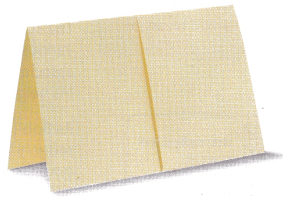# Texas Go Math Grade 8 Module 14 Answer Key Scatter Plots

Refer to our Texas Go Math Grade 8 Answer Key Pdf to score good marks in the exams. Test yourself by practicing the problems from Texas Go Math Grade 8 Module 14 Answer Key Scatter Plots.

## Texas Go Math Grade 8 Module 14 Answer Key Scatter Plots

Evaluate each expression for the given value of x.

Question 1.
6x – 5 for x = 4
Substitute the given value for x
6x – 5 = 6(4) – 5
= 24 – 5
= 19

Question 2.
-2x + 7 for x = 2
Substitute the given value for x
-2x + 7 = -2(2) + 7
= -4 + 7
= 3

5x – 6 for x = 3
Substitute the given value for x
5x – 6 = 5(3) – 6
= 15 – 6
= 9

Question 4.
0.5x + 8.4 for x = -1
Substitute the given value for x
0.5x + 8.4 = 0.5(-1) + 8.4
= -0.5 + 8.4
= 7.9

Question 5.
$$\frac{3}{4}$$x – 9 for x = -20
Substitute the given value for x
$$\frac{3}{4}$$x – 9 = $$\frac{3}{4}$$(-20) – 9
= -15 – 9
= -24

Question 6.
1.4x + 3.5 for x = -4
Substitute the given value for x
1.4x + 3.5 = 1.4(-4) + 3.5
= -5.6 + 3.5
= -2.1

Solve for x.

Question 7.
3x + 4 = 10
3x + 4 = 10
-4 = -4 Subtract 4 from both sides.
3x = 6
$$\frac{3 x}{3}=\frac{6}{3}$$ Divide both sides by 3
x = 2

Question 8.
5x – 11 = 34
5x – 11 = 34
+11 = +11 Add 11 from both sides.
5x = 45
$$\frac{5 x}{5}=\frac{45}{5}$$ Divide both sides by 5.
x = 9

Question 9.
-2x + 5 = -9
-2x + 5 = -9
-5 = -5 Subtract 5 from both sides.
-2x = -14
$$\frac{-2 x}{-2}=\frac{-14}{-2}$$ Divide both sides by -2.
x = 7

Go Math Grade 8 Answer Key Pdf Module 14 Scatter Plots Question 10.
8x + 13 = -11
8x + 13 = 11
-13 = -13 Subtract 13 from both sides.
8x = 24
$$\frac{8 x}{8}=\frac{-24}{8}$$ Divide both sides by 8.
x = -3

Question 11.
4x – 7 = -27
4x – 7 = 27
+7 = +7 Add 7 to both sides
4x = 20
$$\frac{4 x}{4}=\frac{-20}{4}$$ Divide both sides by 4.
x = -5

Question 12.
$$\frac{1}{2}$$x + 16 = 39
$$\frac{1}{2}$$x + 16 = 39
-16 = – 16 Subtract 16 from both sides.
$$\frac{1}{2}$$x = 23
$$\frac{1}{2}$$x ∙ 2 = 23 ∙ 2 Multiply both sides by 2.
x = 46

Question 13.
$$\frac{2}{3}$$x – 16 = 12
$$\frac{2}{3}$$x – 16 + 16 = 12 + 16 Add 16 to both sides
$$\frac{2}{3}$$x = 28

$$\frac{2}{3}$$x × $$\frac{3}{2}$$ = 28 × $$\frac{3}{2}$$ Multiply both sides by the reciprocal of $$\frac{2}{3}$$, which is $$\frac{3}{2}$$.
x = $$\frac{3 \cdot 28}{2}$$

x = $$\frac{3 \cdot 28}{2}$$ Multiply the numerator.
x = $$\frac{84}{2}$$

x = $$\frac{84}{2}$$ Divide
x = 42

Module 14 Scatter Plots Answer Key Go Math Grade 8 Question 14.
0.5x – 1.5 = -6.5
0.5x – 1.5 = -6.5
+1.5 = +1.5 Add 1.5 to both sides.
0.5x = 5
$$\frac{0.5 x}{0.5}=\frac{-5}{0.5}$$ Divide both sides by 0.5.
x = – 10

Visualize Vocabulary

Use the ✓ words to complete the right column of the chart.Understand Vocabulary

Match the term on the left to the correct expression on the right.

1. cluster – A. A data point that is very different from the rest of the data in a set
2. outlier – B. A straight line that comes closest to the points on a scatter plot.
3. trend line – C. A set of closely grouped data.